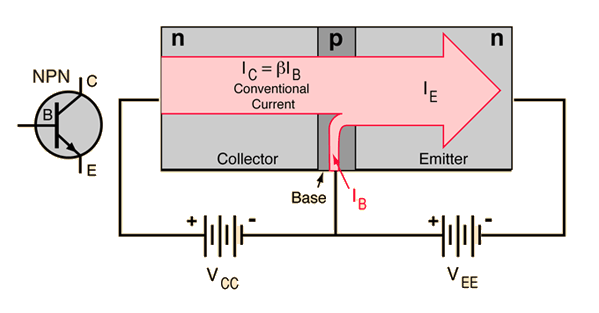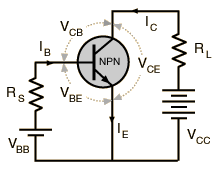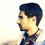# Common Emitter BJT Mechanism

In the active region of a bipolar junction transistor (BJT), the base-emitter junction is forward biased and the base-collector junction is reverse biased.

The common base BJTs do this biasing across the two p-n junctions (base-emitter and base-collector) using two different source. [ $V_{CC}$ and $V_{EE}$ in this case.]These two sources control two different junctions. Or in other words, any one particular source doesn't have control over both the p-n junctions.

But, this is not the case in common emitter configuration.Like you see in the above picture, $V_{CC}$ makes the base-emitter junction forward biased as well as $V_{BB}$.

If we want the common emitter BJTs to conduct current, can't we just apply $V_{CC}$ only? What's the use of $V_{BB}$ here anyway? Isn't it kind of redundant?

# ElectronicsNote by Atomsky Jahid
4 years, 4 months ago

This discussion board is a place to discuss our Daily Challenges and the math and science related to those challenges. Explanations are more than just a solution — they should explain the steps and thinking strategies that you used to obtain the solution. Comments should further the discussion of math and science.

When posting on Brilliant:

• Use the emojis to react to an explanation, whether you're congratulating a job well done , or just really confused .
• Ask specific questions about the challenge or the steps in somebody's explanation. Well-posed questions can add a lot to the discussion, but posting "I don't understand!" doesn't help anyone.
• Try to contribute something new to the discussion, whether it is an extension, generalization or other idea related to the challenge.
• Stay on topic — we're all here to learn more about math and science, not to hear about your favorite get-rich-quick scheme or current world events.

MarkdownAppears as
*italics* or _italics_ italics
**bold** or __bold__ bold
- bulleted- list
• bulleted
• list
1. numbered2. list
1. numbered
2. list
Note: you must add a full line of space before and after lists for them to show up correctly
paragraph 1paragraph 2

paragraph 1

paragraph 2

[example link](https://brilliant.org)example link
> This is a quote
This is a quote
    # I indented these lines
# 4 spaces, and now they show
# up as a code block.

print "hello world"
# I indented these lines
# 4 spaces, and now they show
# up as a code block.

print "hello world"
MathAppears as
Remember to wrap math in $$ ... $$ or $ ... $ to ensure proper formatting.
2 \times 3 $2 \times 3$
2^{34} $2^{34}$
a_{i-1} $a_{i-1}$
\frac{2}{3} $\frac{2}{3}$
\sqrt{2} $\sqrt{2}$
\sum_{i=1}^3 $\sum_{i=1}^3$
\sin \theta $\sin \theta$
\boxed{123} $\boxed{123}$

## Comments

Sort by:

Top Newest

Actually VBB is not at all useless! Often varible VCC is used, and in those cases when VCC becomes zero we still need more negativity in the emitter terminal than the base. That requirement is then fulfilled by the source VBB.

- 4 years, 4 months ago

Log in to reply

Assuming the resistances are small enough, you can think of VBB as controlling the base emitter junction, while VCC - VBB controls the base collector junction. The two most common uses for the common emitter configuration are switching (1s and 0s) and signal amplification. For switching, we can apply VBB = VCC so that the BJT conducts (ON, saturation region), and VBB = 0 so that the BJT does not conduct (OFF, cutoff region). For signal amplification, VBB is chosen such that the BJT is in the active region, which you can think of as something in between ON and OFF. You can actually "make a VBB" out of VCC by using a voltage divider.

- 4 years, 4 months ago

Log in to reply

Actually, $V_{CC}$ doesn’t have much effect on the base-emitter junction directly. Consider putting two (normal, not Zener) diodes in series face-to-face in a circuit. Now, what’s the voltage drop across the two diodes?Simulated Circuit

[I've tried to embed the photo a few times. But, it isn't working. Try this link: "https://goo.gl/photos/23uusj2DJMBcKbrWA".]

D1 (the diode which is reverse biased) exhaust almost all of the voltage supply. On the other hand, the voltage drop across D2 (the diode which is forward biased) is very small, $26 \times 10^{-3}$ V to be precise.

The scenario in common emitter BJT is similar (kind of). $V_{CE}$ (which is a portion of $V_{CC}$) is not enough to overcome the base-emitter junction barrier voltage because almost all of it will be dropped against the collector-base junction. That’s why $V_{BB}$ is needed to provide the necessary $V_{BE}$ which makes the conduction of current possible.

- 4 years, 4 months ago

Log in to reply

×

Problem Loading...

Note Loading...

Set Loading...